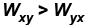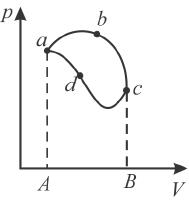Courses

# First Law Of Thermodynamics MCQ Level – 1 (part - 2)

## 10 Questions MCQ Test Topic wise Tests for IIT JAM Physics | First Law Of Thermodynamics MCQ Level – 1 (part - 2)

Description
This mock test of First Law Of Thermodynamics MCQ Level – 1 (part - 2) for IIT JAM helps you for every IIT JAM entrance exam. This contains 10 Multiple Choice Questions for IIT JAM First Law Of Thermodynamics MCQ Level – 1 (part - 2) (mcq) to study with solutions a complete question bank. The solved questions answers in this First Law Of Thermodynamics MCQ Level – 1 (part - 2) quiz give you a good mix of easy questions and tough questions. IIT JAM students definitely take this First Law Of Thermodynamics MCQ Level – 1 (part - 2) exercise for a better result in the exam. You can find other First Law Of Thermodynamics MCQ Level – 1 (part - 2) extra questions, long questions & short questions for IIT JAM on EduRev as well by searching above.
QUESTION: 1

### If W is the work done by a system against its surrounding, what will –W stand for ? Select one:

Solution:

If W is work done by the system then –W would be the work done on the system by its surroundings.
The correct answer is: Work done on the system by its surroundings

QUESTION: 2

### Internal energy of a perfect gas depends on. Select one:

Solution:

dU = nCvdT.
The correct answer is: temperature only

QUESTION: 3

### In a cyclic process. Select one:

Solution:

Explanation : In a cyclic process, the system starts and returns to the same thermodynamic state.

Thus, change in internal energy in a cyclic process  ΔU=0

From !st law of thermodynamics,  ΔQ = ΔU + ΔW

⟹ ΔQ = 0 + ΔW

ΔQ = ΔW

QUESTION: 4

Which of the following is not a property of the system?
Select one:

Solution:

Heat is thermal energy transferred from a hotter body to a cooler body that arein contact. Hence, it is not a property of the system.

QUESTION: 5

If a system A is in thermal equilibrium separately with B and C, then B and C are also in thermal equilibrium with each other. This is the statement for.
Select one:

Solution:

The above statement is nothing but zeroth law of thermodynamics.
The correct answer is: Zeroth law of thermodynamics

QUESTION: 6

The processes or systems that do not involve heat is called.
Select one:

Solution:

In adiabatic processes, dQ = 0 heat remains fixed in the process, hence adiabatic processes do not involve heat.

QUESTION: 7

Let ΔWi = amount of work done by the gas when compressed isothermally to volume V, ΔQ = amount of heat absorbed by the gas during the process, ΔWa = amount of work done by the gas when expanded adiabatically to volume V, ΔE = change in internal energy of the gas due to complete process. So ΔE is given by.
Select one:

Solution:

Solution= C

dU = dQ – dW

⇒ ΔU = ΔQ – ΔW

ΔW= ΔWi-ΔWa

ΔW, work done by gas when compressed isothermally is positive.

dU = dQ – (ΔdWi-ΔWa)

=ΔQ- ΔdWi-ΔWa

Hence the correct answer would be ΔQ- ΔWi-ΔWa

QUESTION: 8

First law of the thermodynamics is conservation of.
Select one:

Solution:

dθ = dU + dW

QUESTION: 9

The piston containing an ideal gas is originally is the state x (see figure). The gas is taken through a thermal cycle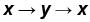as shown.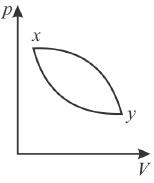The work done by the gas is positive, if the direction of the thermal cycle is
Select one:

Solution:

Since work done = Area between p-V curve and volume axis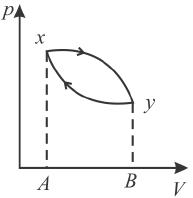Hence, work in positive direction is clockwise
Wxy = Area xyBA
Wyx = Area yBAx
and